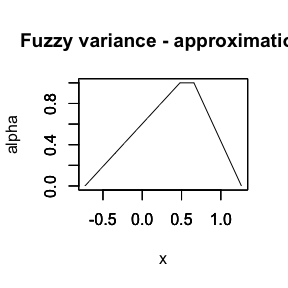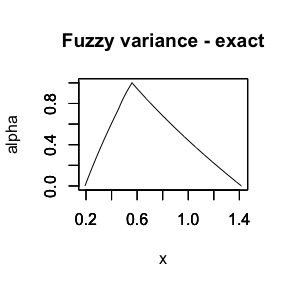On statistical measures

library(FuzzySTs)

Fuzzy.sample.mean(): Calculates the fuzzy sample mean

FuzzySTs::Fuzzy.sample.mean() calculates the fuzzy sample mean of a given fuzzy variable. If the variable is encoded by trapezoidal fuzzy numbers written by their quadruple, this function can return a fuzzy number of the same form. The function can return as well a fuzzy number given by its numerical $$\alpha$$-cuts.

Practically, if the parameter alphacuts="TRUE", the function returns a matrix composed by 2 vectors representing the numerical left and right $$\alpha$$-cuts. For this output, the function FuzzySTs::is.alphacuts() returns a value TRUE. If the parameter alphacuts="FALSE", FuzzySTs::Fuzzy.sample.mean() returns a trapezoidal fuzzy number given by the quadruple (p,q,r,s).

# Simple example
mat <- matrix(c(1,2,2,3,3,4,4,5), ncol =4)
Fuzzy.sample.mean(mat)
#> Trapezoidal fuzzy number with:
#>    support=[1.5,4.5],
#>       core=[2.5,3.5].
is.alphacuts(mat)
#>  FALSE

Weighted.fuzzy.mean(): Calculates the weighted fuzzy sample mean

FuzzySTs::Weighted.fuzzy.mean() calculates the weighted fuzzy sample mean of a given fuzzy variable. If the variable is encoded by trapezoidal fuzzy numbers written by their quadruple, this function can return a fuzzy number of the same form. The function can return as well a fuzzy number given by its numerical $$\alpha$$-cuts.

Practically, if the parameter alphacuts="TRUE", the function returns a matrix composed by 2 vectors representing the numerical left and right $$\alpha$$-cuts. For this output, the function FuzzySTs::is.alphacuts() returns a value TRUE. If the parameter alphacuts="FALSE", FuzzySTs::Fuzzy.sample.mean() returns a trapezoidal fuzzy number given by the quadruple (p,q,r,s).

# Simple example
mat <- matrix(c(1,2,2,3,3,4,4,5), ncol =4)
w <- c(1,3)
Weighted.fuzzy.mean(mat, w)
#> Trapezoidal fuzzy number with:
#>    support=[1.75,4.75],
#>       core=[2.75,3.75].

Moment(): Calculates a central sample moment of a random fuzzy variable

FuzzySTs::Moment() calculates the $$k$$-th classical central sample moment of a random fuzzy variable by the Féron - Puri and Ralescu approach. This moment can be calculated using a distance chosen from the family of distances shown for the function FuzzySTs::distance(). By the function FuzzySTs::Moment(), one can easily compute the skewness and the kurtosis measures of the considered random fuzzy variable.

# Simple example
mat <- matrix(c(1,2,2,3,3,4,4,5), ncol =4)
Moment(mat, k=4, dist.type = "GSGD")
#>  0.125

Skewness(): Calculates the skewness of a random fuzzy variable

FuzzySTs::Skewness() calculates the skewness of a random fuzzy variable based on the expression of the classical central sample moments. The calculations are made using the function FuzzySTs::Moment(). For a random fuzzy variable $$\tilde{X}$$, the skewness is given by the following ratio: $\begin{equation} \text{Skewness} (\tilde{X}) = \frac{\nu_3(\tilde X)}{(\nu_2(\tilde X))^{3/2}}, \end{equation}$ where $$\nu_3(\tilde X)$$ is the third central sample moment of the variable $$\tilde{X}$$, and $$\nu_2(\tilde X)$$ is its second central sample moment.

# Simple example
mat <- matrix(c(1,2,0.25,1.8,2,2.6,0.5,3,3,2.6,3.8,4,4,4.2,3.9,5), ncol =4)
Skewness(mat, dist.type = "GSGD")
#>  -0.154327

Kurtosis(): Calculates the excess of kurtosis of a random fuzzy variable

FuzzySTs::Kurtosis() calculates the excess of kurtosis of a random fuzzy variable based on the expression of the classical central sample moments. The calculations are made using the function FuzzySTs::Moment(). For a random fuzzy variable $$\tilde{X}$$, the excess of kurtosis is given by the following ratio: $\begin{equation} \text{Kurtosis} (\tilde{X}) = \frac{\nu_4(\tilde X)}{(\nu_2(\tilde X))^{2}} - 3, \end{equation}$ where $$\nu_4(\tilde X)$$ is the fourth central sample moment of the variable $$\tilde{X}$$, and $$\nu_2(\tilde X)$$ is its second central sample moment.

# Simple example
mat <- matrix(c(1,2,0.25,1.8,2,2.6,0.5,3,3,2.6,3.8,4,4,4.2,3.9,5), ncol =4)
Kurtosis(mat, dist.type = "GSGD")
#>  -1.93011

Fuzzy.variance(): Calculates the variance of a fuzzy variable

FuzzySTs::Fuzzy.variance() calculates the variance of a fuzzy variable. By this function, one could compute the following types of variances:

• the Fréchet variance of a random fuzzy variable.

• a numerical “point to point” fuzzy type variance for trapezoidal and triangular fuzzy numbers using the functions FuzzySTs::Fuzzy.Difference() and FuzzySTs::Fuzzy.Square().

We have to mention that for the calculations of this fuzzy variance, any type of fuzzy numbers can be used. However, these numbers will be treated as trapezoidal fuzzy numbers. A condition on the monotony of the left of the right $$\alpha$$-level sets of the produced fuzzy number is postulated. Thus, the left side should always be ascending, and the right one should always be descending.

For the fuzzy variance by this method, we propose two additional functions defined as $\texttt{Fuzzy.exact.variance.poly.left (object, breakpoints = 100)}$ $\text{and } \texttt{Fuzzy.exact.variance.poly.right (object, breakpoints = 100)},$ which produce the polynomial forms of the numerical $$\alpha$$-cuts obtained using the function $\texttt{Fuzzy.variance (object, method="exact", \ldots)}.$

The output is a table composed by the coefficients of the second order equations of the left and the right sides, given at the corresponding definitions domains.

• a cheap fuzzy type variance basically for trapezoidal and triangular fuzzy numbers by $$5$$ different approximations of the fuzzy square. Note that for these computations, the function FuzzySTs::Fuzzy.Difference() is used. We add that the fuzzification matrix should be obtained by the function FuzzySTs::FUZZ() for trapezoidal and triangular fuzzy numbers. The different approximations can be written as follows:

• Approximation $$1$$: For a trapezoidal fuzzy number $$\tilde{X}=(p,q,r,s)$$, $$p\leq q \leq r \leq s$$, the fuzzy square of $$\tilde{X}$$ can be approximated by: $\tilde{X} \otimes \tilde{X} = (p,q,r,s) \otimes (p,q,r,s) =\big(q^2 - 2qp, q^2, r^2, 2rs - r^2\big).$
• Approximation $$2$$: For a trapezoidal fuzzy number $$\tilde{X}=(p,q,r,s)$$, $$p\leq q \leq r \leq s$$, the fuzzy square of $$\tilde{X}$$ can be approximated by: $\tilde{X} \otimes \tilde{X} = (p,q,r,s) \otimes (p,q,r,s) = \big(\min (p^2,ps), q^2, r^2, \max (ps,s^2)\big).$
• Approximation $$3$$: Consider a trapezoidal fuzzy number $$\tilde{X}=(p,q,r,s)$$, $$p\leq q \leq r \leq s$$. Let $$T(\tilde{X})=(p_r,q_r,r_r,s_r)$$ be the trapezoidal fuzzy number resulting from the approximation of $$\tilde{Y} = \tilde{X} \otimes \tilde{X}$$. The quadruple representing $$T(\tilde X)$$ is given by the following: $\begin{eqnarray} p_r = \max \big\{ y \in \tilde{Y}^{\alpha}_L \mid \mu_{\tilde{X} \otimes \tilde{X}} (y) = 0 \big\}, \notag \\ q_r = \min \big\{ y \in \tilde{Y}^{\alpha}_L \mid \mu_{\tilde{X} \otimes \tilde{X}} (y) = 1 \big\}, \notag \\ r_r = \max \big\{ y \in \tilde{Y}^{\alpha}_R \mid \mu_{\tilde{X} \otimes \tilde{X}} (y) = 1 \big\}, \notag \\ s_r = \min \big\{ y \in \tilde{Y}^{\alpha}_R \mid \mu_{\tilde{X} \otimes \tilde{X}} (y) = 0 \big\}. \notag \end{eqnarray}$
• Approximation $$4$$: For a fuzzy number defined as a piecewise one, we approximate its fuzzy square using the product operation $$*$$ defined by the FuzzyNumbers package.
• Approximation $$5$$: For a trapezoidal fuzzy number $$\tilde{X}=(p,q,r,s)$$, $$p\leq q \leq r \leq s$$, the fuzzy square of $$\tilde{X}$$ can be approximated by: $\begin{eqnarray*} \tilde{X} \otimes \tilde{X} &=& (p,q,r,s) \otimes (p,q,r,s) \\ &=& \Big( \min (p^2,ps,s^2), \min (q^2, qr, r^2) ,\max (q^2, qr, r^2), \max (p^2,ps,s^2) \Big). \end{eqnarray*}$

Using almost all of these approximations, a computational complexity induced by the approximation operation is expected to occur. It is related to the ordering of the obtained non-positive elements of the quadruples defining the fuzzy numbers. This fact violates the principles of the direction of the left and right $$\alpha$$-cuts of a LR fuzzy number. Therefore, we proposed to solve the problem using the shifting technique, also known as the translation technique.

To sum up, in terms of outcome, if the parameter method = "distance", the function FuzzySTs::Fuzzy.variance() returns a numerical value. If else, it returns the numerical $$\alpha$$-cuts of the estimated fuzzy variance by one of the approximation methods chosen.

# Example 1
data <- matrix(c(1,2,3,2,2,1,1,3,1,2),ncol=1)
MF111 <- TrapezoidalFuzzyNumber(0,1,1,2)
MF112 <- TrapezoidalFuzzyNumber(1,2,2,3)
MF113 <- TrapezoidalFuzzyNumber(2,3,3,3)
PA11 <- c(1,2,3)

# Fuzzification using FUZZ giving a matrix of the quadruples p,q,r,s

data.fuzzified <- FUZZ(data,mi=1,si=1,PA=PA11)
Fuzzy.variance(data.fuzzified, method = "approximation5", plot=TRUE)#> Trapezoidal fuzzy number with:
#>    support=[-0.728889,1.26222],
#>       core=[0.48,0.657778].#>              L        U
#> 0.00 0.1920000 1.416000
#> 0.01 0.1950464 1.405935
#> 0.02 0.1981056 1.395901
#> 0.03 0.2011776 1.385897
#> 0.04 0.2042624 1.375923
#> 0.05 0.2073600 1.365980
Fuzzy.variance(data.fuzzified, method = "distance")
#>  0.5
# Example 2 - Fuzzification using GFUZZ giving a numerical matrix of left and right alpha-cuts

data.fuzzified2 <- GFUZZ(data,mi=1,si=1,PA=PA11)